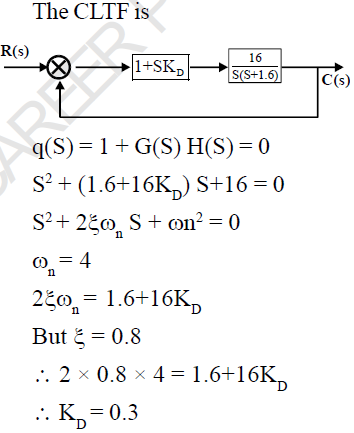Courses

# Control System - MCQ Test 3

## 25 Questions MCQ Test Mock Test Series for Electrical Engineering (EE) GATE 2020 | Control System - MCQ Test 3

Description
This mock test of Control System - MCQ Test 3 for GATE helps you for every GATE entrance exam. This contains 25 Multiple Choice Questions for GATE Control System - MCQ Test 3 (mcq) to study with solutions a complete question bank. The solved questions answers in this Control System - MCQ Test 3 quiz give you a good mix of easy questions and tough questions. GATE students definitely take this Control System - MCQ Test 3 exercise for a better result in the exam. You can find other Control System - MCQ Test 3 extra questions, long questions & short questions for GATE on EduRev as well by searching above.
QUESTION: 1

### The OLTF of closed loop system is given by the following Transfer function.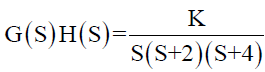The maximum value of K for which the system just become unstable is-

Solution: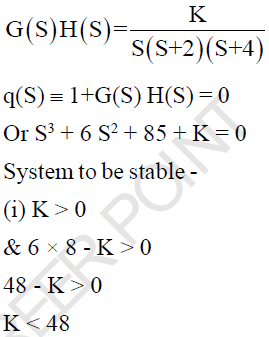QUESTION: 2

Solution:
QUESTION: 3

### The control system shown in the given fig has an internal rate Feedback loop. The closed-loop system for open and closed condition of the switch will be respectively-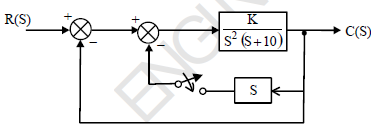Solution: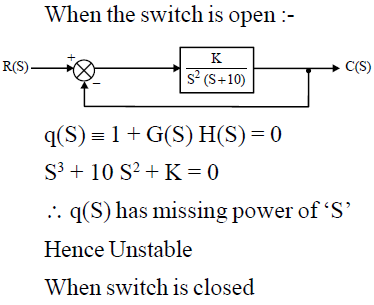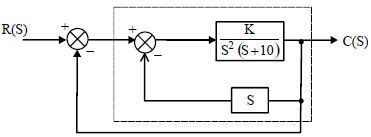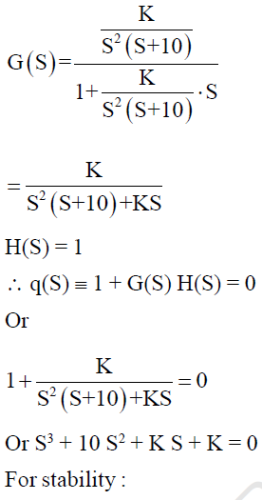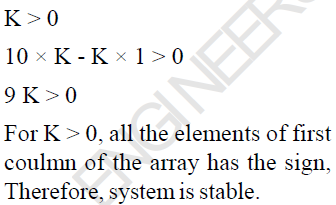*Answer can only contain numeric values
QUESTION: 4

An under damped second order  system having Transfer function of the form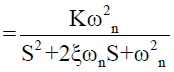has a frequency response shown below, The system gain ‘K’ is...?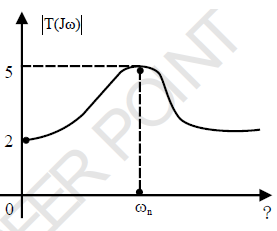Solution: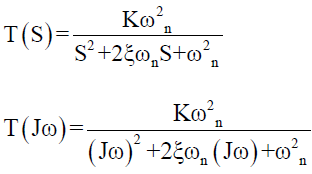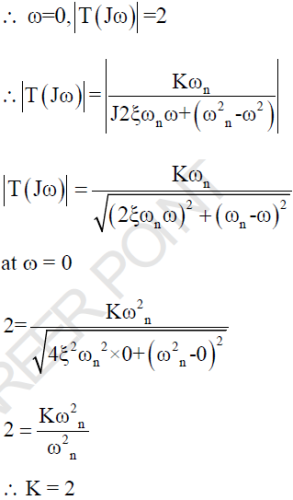QUESTION: 5

The OLTF of -ve UFB control system is given by-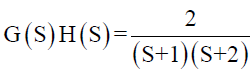The gain margin (in dB) is given by-

Solution: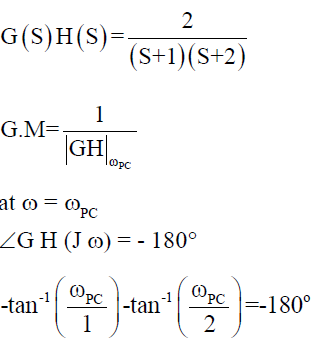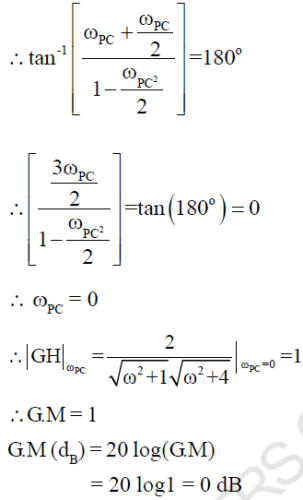QUESTION: 6

A unity Feed back system has forword loop gain T.F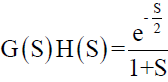. The cross over frequency (ωPC) is given by -

Solution: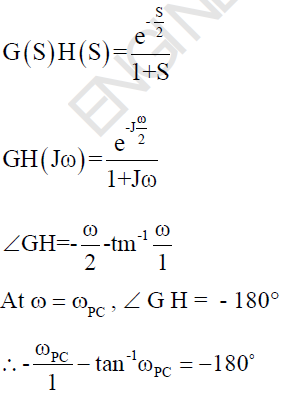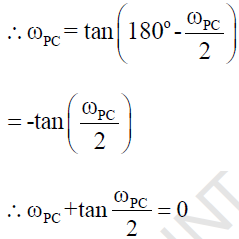QUESTION: 7

The Magnitude Bode Plot of a certain system is shown below.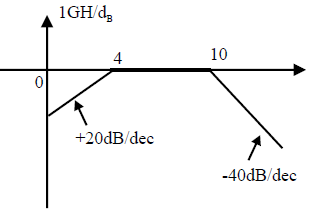What will be its Transfer function..?

Solution: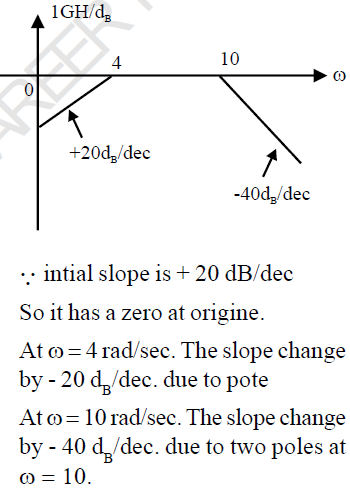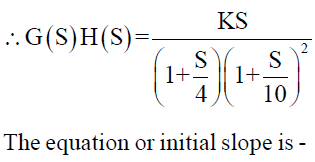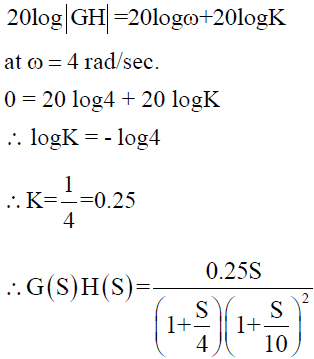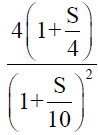QUESTION: 8

The T.F. of control system is given by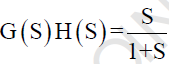. The polar plot of the system lies in-

Solution: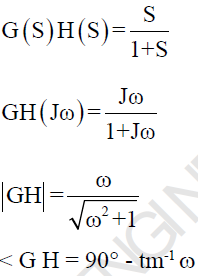QUESTION: 9

A bolock diagram is shown in below.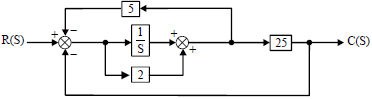The closed loop poles of this system are.

Solution: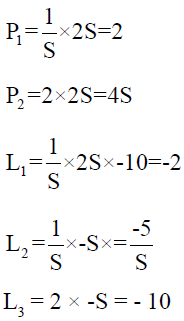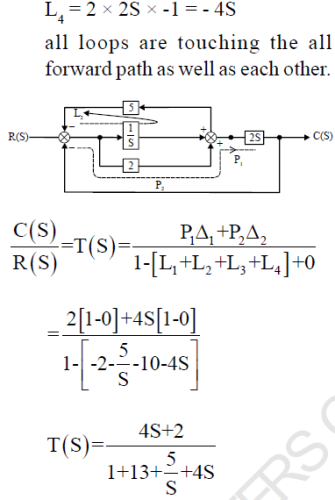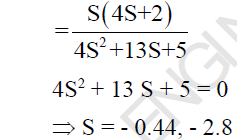QUESTION: 10

The response C(t) of a system to an input r(t) is given by the following differential equation: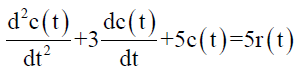The Transfer function of the system is given by-

Solution: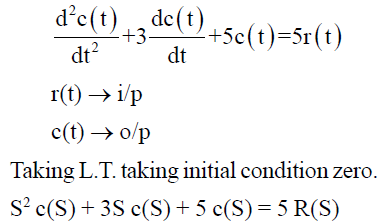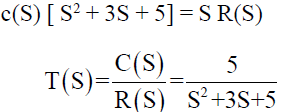QUESTION: 11

A second order system is defined by the following differential equation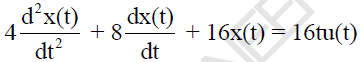The damping ratio and natural prog. For this system is respectively.

Solution: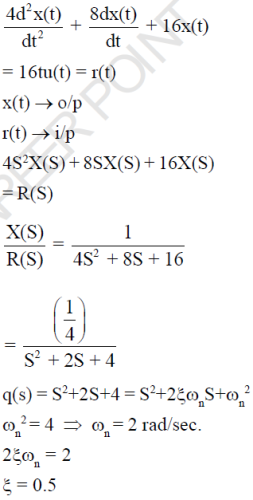QUESTION: 12

For the given system the magnitude of CLTF sensitivity w.r.t OLTF at  frequency ω = 1 rad/sec is ?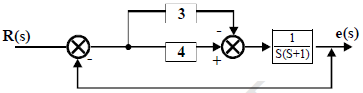Solution: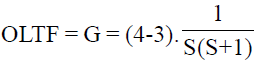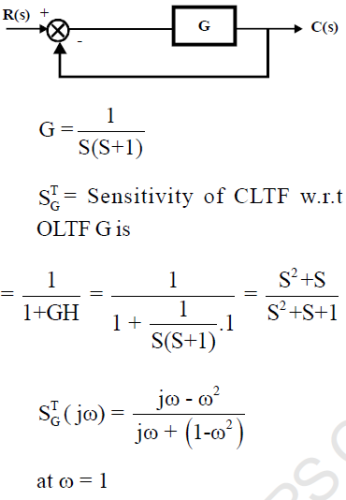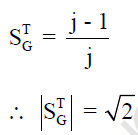QUESTION: 13

Find the impulse response of the system having transfer function each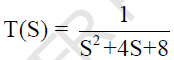Solution: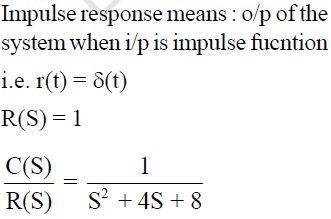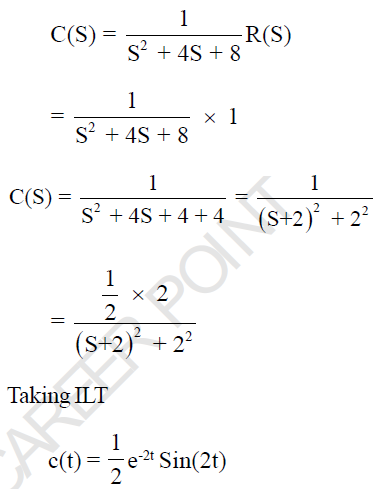QUESTION: 14

A closed loop control system has G(S) = 100/S2 and H(S) = (S+1) The steady state output for a unit step
input is…?

(Important - Enter only the numerical value in the answer)

Solution: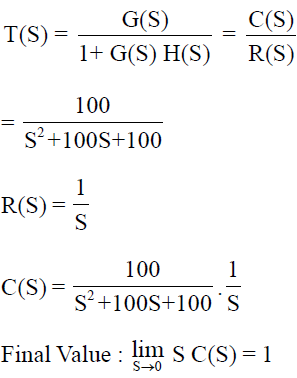QUESTION: 15

A linear time invariant system initially at rest, when subjected to unit step input gives a response y(t) = te-t, t > 0 The corresponding transfer function is

Solution: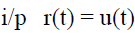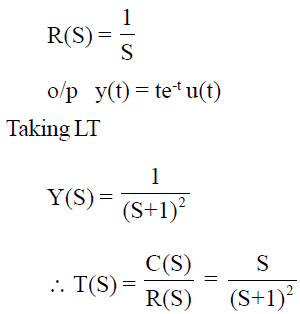QUESTION: 16

The responsse of a system is shown by the given wave-form. The correct root location in the S- Plane is ..?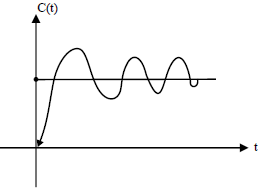Solution:

Given fig is response of the system which is under-damped

i.e. 0<ξ<1

For under-damped system, Poles are Comlex-Conjugate and lies on left half of S-Plane.

*Answer can only contain numeric values
QUESTION: 17

Consider a causal L T I system characterized by the Differential equations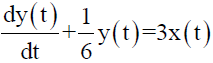The steady state o/p of the system to input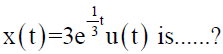(Important - Enter only the numerical value in the answer

Solution: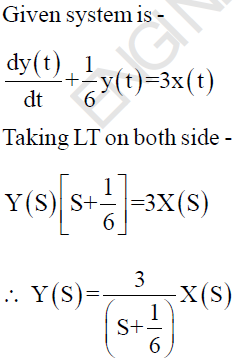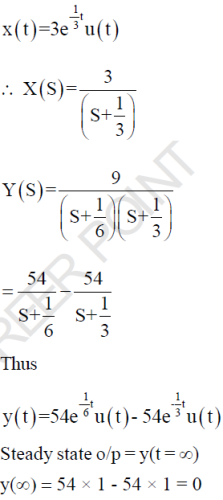QUESTION: 18

The system shown in the given fig. is subjected to a unit rump input.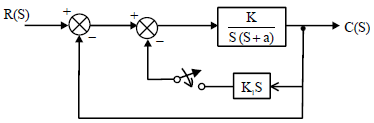On closing the switches

Solution: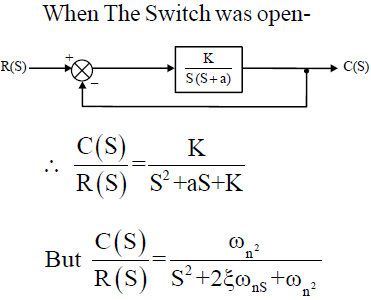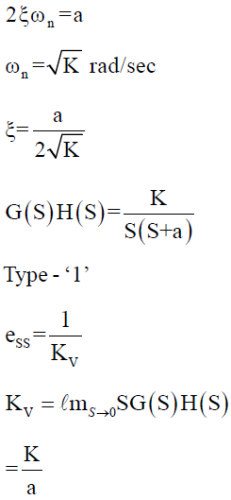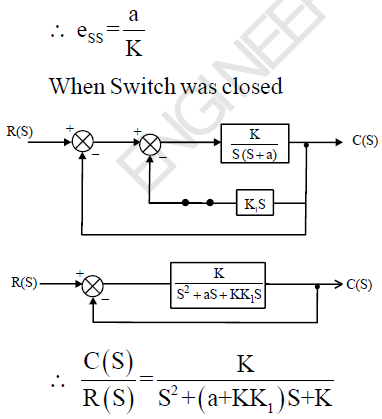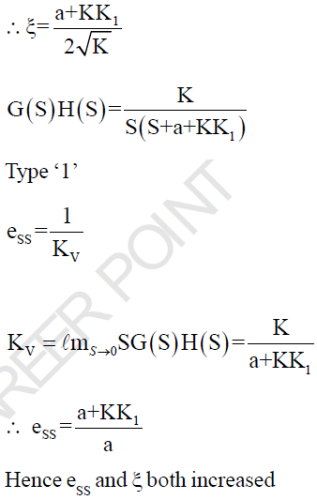QUESTION: 19

The impulse response of an initially relaxed linear system is e-2t , t > 0. To produce a response of t e- 2t , t >0, The input must be equal to -

Solution: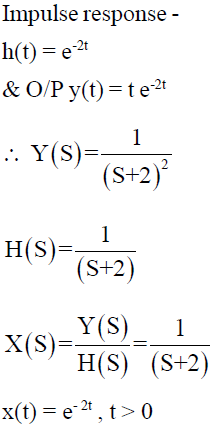QUESTION: 20

A control system is discribed by the following equation -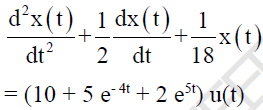The Natural time constants of the system are-

Solution: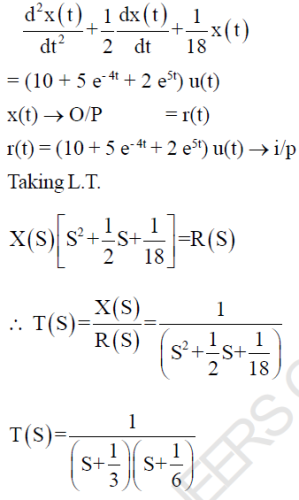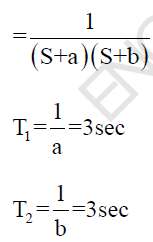QUESTION: 21

The root location for a second order system is shown, the dumping ratio and natural freq. of system is respectively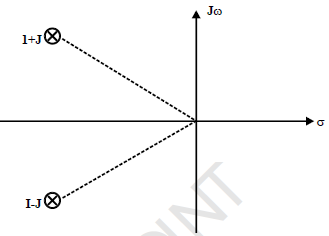Solution: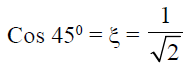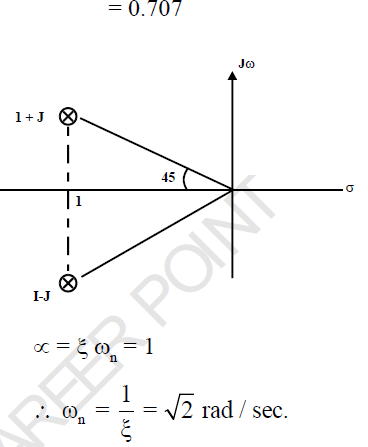QUESTION: 22

Consider UFB system with forward path T.F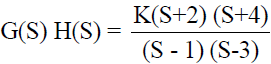The range of K for which system is stable

Solution: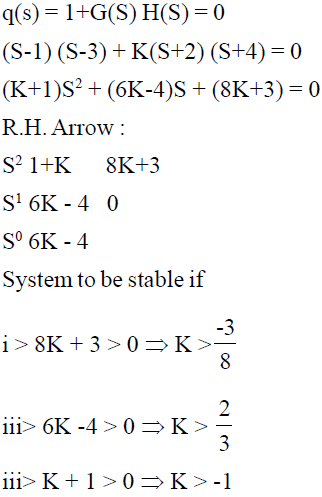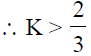*Answer can only contain numeric values
QUESTION: 23

An open loop control system results in a response of

(10e-2t - 3e-t) u(t)

For an unit impulse input. The D.C. gain of control system is….....?

(Important - Enter only the numerical value in the answer)

Solution: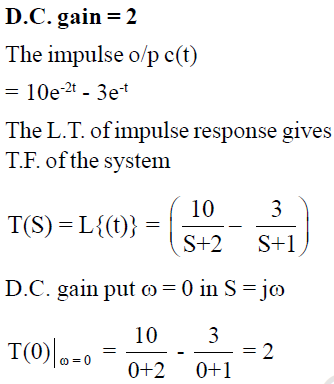QUESTION: 24

For the given system the value of  K and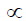so that the system oscillates at freq. ω = 1 rad/sec.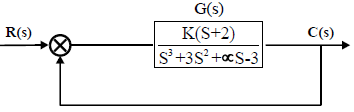(Important - Enter only the numerical value in the answer)

Solution:

K = 3 &= -2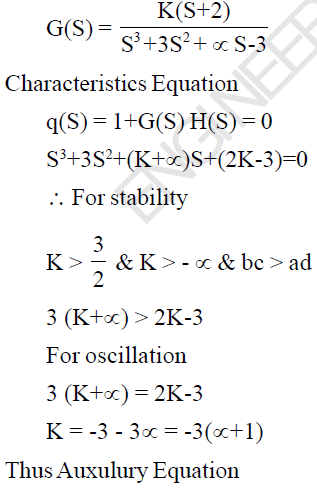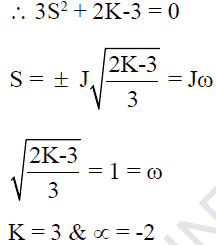*Answer can only contain numeric values
QUESTION: 25

A system with derivative controller is given as below. The value of KD for which the damping ratio becomes 0.8 is ?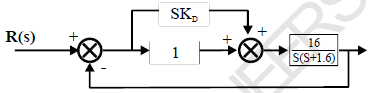(Important - Enter only the numerical value in the answer)

Solution: